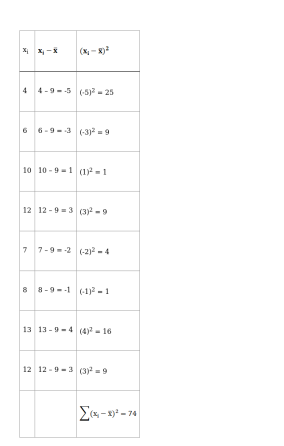# Find the mean, variance and standard deviation for the numbers 4, 6, 10, 12,

Question:

Find the mean, variance and standard deviation for the numbers 4, 6, 10, 12, 7, 8, 13, 12.

Solution:

Given data: 4, 6, 10, 12, 7, 8, 13, 12

To find: MEAN

We know that

Mean $(\overline{\mathrm{x}})=\frac{\text { Sum of observations }}{\text { Total number of observations }}$

$=\frac{4+6+10+12+7+8+13+12}{8}$

$=\frac{72}{8}$

$\overline{\mathrm{x}}=9$

To find: VARIANCEVariance, $\sigma^{2}=\frac{\sum\left(\mathrm{x}_{\mathrm{i}}-\overline{\mathrm{x}}\right)^{2}}{\mathrm{n}}$

$=\frac{74}{8}$

$=9.25$

To find: STANDARD DEVIATION

Standard Deviation $(\sigma)=\sqrt{\text { Variance }}$

$=\sqrt{9.25}$

$=3.04$